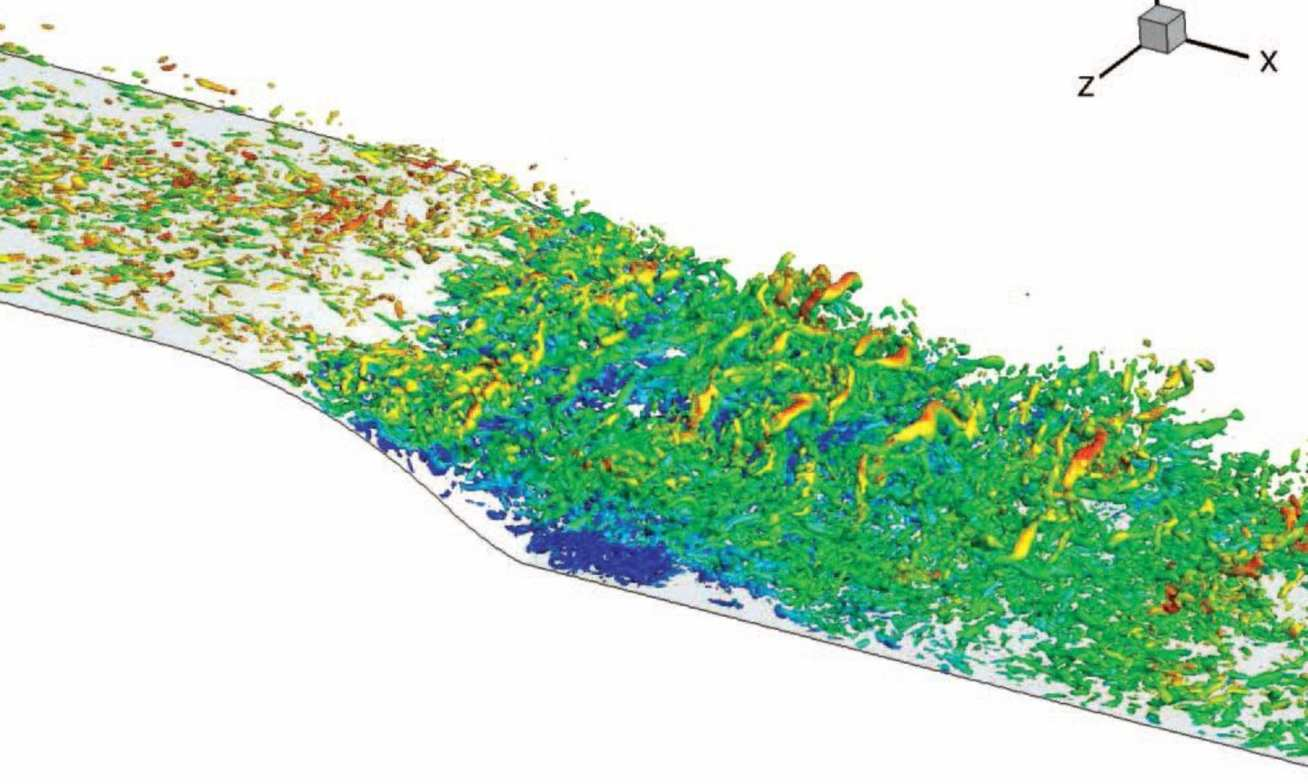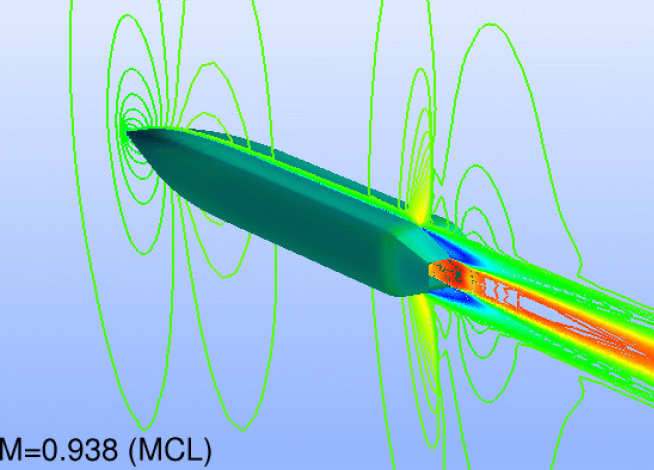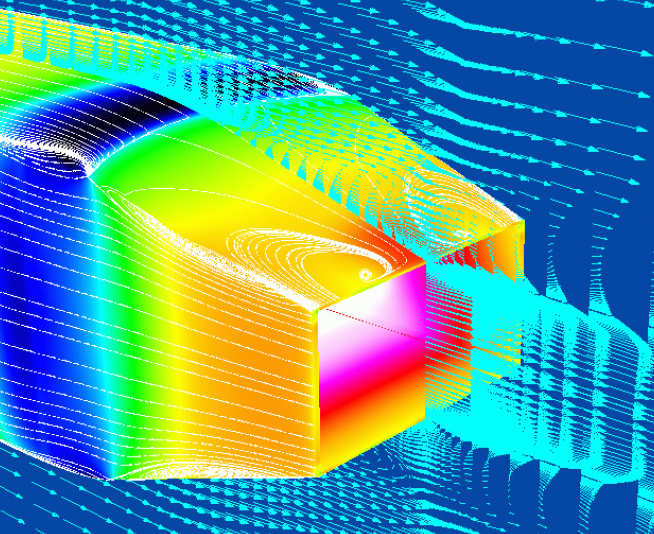# Simulation vs. Modelling

Turbulence simulation, in its purest form – called Direct Numerical Simulation or DNS, aims to resolve all details and scales of the turbulence, i.e. the spatial and temporal evolution of the entire range of eddies. This is an extremely expensive approach, often requiring 108-1010 computational nodes and CPU times extending to millions of CPU hours. The two images below arise from a DNS of a flow in a channel, but at relatively low Reynolds numbers.

At practically relevant Reynolds numbers, the linear size of the smallest turbulent eddies may be only 10-3-10-4 of that of the largest eddies, and DNS is then far too expensive. The solution is then to model the effects of small eddies eddies and only resolve the large, most energetic eddies, the smallest resolved size being of order 10-2 of the appropriate dimension of the flow. This approach is called Large Eddy Simulation and is one of the most important computational tools used by the TFMS Group in its research.Large Eddy Simulation of a separated flow over a curved step (blue=reverse flow)

Turbulence modelling is an alternative to simulation, the latter resolving the time and space evolution of turbulence within a turbulent flow.  Modelling is based on the integration of the flow-governing equations in time, thus yielding equations for the time-averaged flow properties. In this approach, all time-dependent features are assimilated into statistical correlations which arise from time-averaging the Navier-Stokes equations. Any turbulent quantity is decomposed into its mean and a fluctuation. This decomposition is then inserted into the equations, followed by time-averaging. The result is a set of "Reynolds-averaged Navier-Stokes equations" describing the spatial variations of time-mean quantities. The consequence of this integration is the appearance of unknown time-correlations of turbulent velocity fluctuations. These represent, in effect, the influence of turbulence on the distribution of the time-averaged flow properties. To eliminate these correlation, turbulence models are needed. These are statistical closure models, consisting typically of partial differential equations for time-mean turbulence parameters to which the unknown correlations can be related, or equations for the unknown correlations themselves.

Turbulence modelling has a rich history going back to the 19th century – the seminal work of Osborne Reynolds (1842-1912) being an important landmark (see: Jackson, J.D. (1995), "Osborne Reynolds: scientist, engineer and pioneer", Proc. Royal Society London A, 451, pp. 49-86). More than 150 years of research have spawned hundreds of models and model variants, many of which have been subjected to extensive computational validation over the past 35 years by reference to numerous laboratory flows for which high-quality experimental data had been obtained. The large number of models reflects the enormous difficulty of deriving a universal closure. The holy grail of turbulence modelling is a mathematical framework that is universal. There is no such model and none can be derived, because turbulence i s not amenable to closure by a finite hierarchy of equations for correlations of higher and higher order. Even if such equations could be derived, their approximation would always entail errors, and their solution would be practically impossible. The text book on the Homepage of this Website is devoted to the subject of turbulence modelling.

Despite the above uncertainties, turbulence models constitute the mainstay of the large majority of practical computations in the industrial environment, and most proprietary flow software incorporates a range of statistical turbulence models.  If these models are used in an informed manner, and if no unsteady data are needed (e.g. on noise or vibrations), the reward of using turbulence models is an ability to solve flow problems at a tiny fraction of the cost of performing corresponding full simulations.Overall view of jet-afterbody flow (Mach contours), with shock wave visible on the afterbody

The images below were obtained from a computation with a "Reynolds-stress-transport model of turbulence".  This consists of differential transport equations for all 6 independed components of the Reynolds-stress tensor and an additional equation for the rate of turbulence dissipation.  The numerical code used wasa higher-order Riemann solver specifically designed for highly compressible flows.  Such a flow cannot currently be computed with DNS or even LES, because of its high Reynolds number and the importance of resolving accurately the boundary layers on the body - a formidable challenge for scale-resolving simulations.Transonic separated flow over jet afterbody, with supersonic jet, computed with a Reynolds-stress-transpot model of turbulence